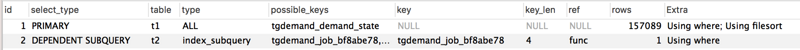### Mysql关系型数据库管理系统

MySQL是一个开放源码的小型关联式数据库管理系统，开发者为瑞典MySQL AB公司。MySQL被广泛地应用在Internet上的中小型网站中。由于其体积小、速度快、总体拥有成本低，尤其是开放源码这一特点，许多中小型网站为了降低网站总体拥有成本而选择了MySQL作为网站数据库。

# 定位原因

```SELECT * FROM tgdemand_demand t1
WHERE
(
t1.id IN
(
SELECT t2.demand_id
FROM tgdemand_job t2
WHERE (t2.state = 'working' AND t2.wangwang = 'abc')
)
AND
NOT (t1.state = 'needConfirm')
)
ORDER BY t1.create_date DESC``````SELECT * FROM tgdemand_demand t1 WHERE EXISTS (
SELECT * FROM tgdemand_job t2 WHERE t1.id = t2.demand_id AND (t2.state = 'working' AND t2.wangwang = 'abc')
) AND NOT (t1.state = 'needConfirm')
ORDER BY t1.create_date DESC;```

# 问题修复

```ids = SELECT t2.demand_id
FROM tgdemand_job t2
WHERE (t2.state = 'working' AND t2.wangwang = 'abc');

SELECT * FROM tgdemand_demand t1
WHERE
(
t1.id IN ids
AND
NOT (t1.state = 'needConfirm')
)
ORDER BY t1.create_date DESC;```

```demand_ids = Job.objects.filter(wangwang=user['wangwang'], state='working').values_list("demand_id", flat=True)

demands = Demand.objects.filter(id__in=demand_ids).exclude(state__in=['needConfirm']).order_by('-create_date')```

```demand_ids = list(Job.objects.filter(wangwang=user['wangwang'], state='working').values_list("demand_id", flat=True))

demands = Demand.objects.filter(id__in=demand_ids).exclude(state__in=['needConfirm']).order_by('-create_date')```

```select * from tgdemand_demand t1, (select t.demand_id from tgdemand_job t where t.state = 'working' and t.wangwang = 'abc') t2
where t1.id=t2.demand_id and not (t1.state = 'needConfirm')
order by t1.create_date DESC```

# 参考资料

MySQL的慢日志线上问题及优化方案
MySQL的分页优化

MySQL的增删查改语句用法示例总结
101个MySQL的配置和优化的提示
mysql 开启慢查询 如何打开mysql的慢查询日志记录